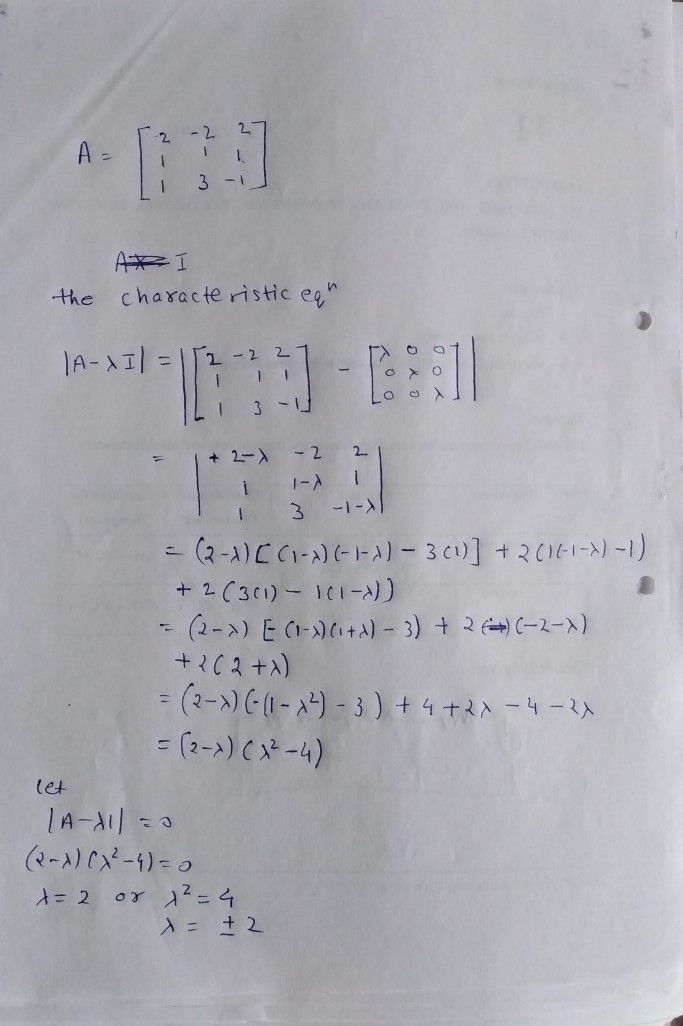Symbol
ProblemEigen value. $-2$ $\left(r2$ 2 is an Eigen value of the matrix $2$ $A=1$ $1$ $1$ $2$ $1$ $-1$ find the other two Eigen valu $3$ 3
Others
SolutionQanda teacher - vschauhan